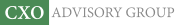Objective research to aid investing decisions

#### Value Investing Strategy (Strategy Overview)

Allocations for December 2023 (Final)
Cash TLT LQD SPY

#### Momentum Investing Strategy (Strategy Overview)

Allocations for December 2023 (Final)
1st ETF 2nd ETF 3rd ETF

# When Rebalancing Works?

| | Posted in: Strategic Allocation, Volatility Effects

Under what conditions is periodic rebalancing a successful “volatility harvesting” strategy? In his February 2014 paper entitled “Disentangling Rebalancing Return”, Winfried Hallerbach analyzes the return from periodic portfolio rebalancing by decomposing its effects into a volatility return and a dispersion discount. He defines:

• Rebalancing return as the difference in (geometric) growth rates between periodically rebalanced and buy-and-hold portfolios.
• Volatility return as the difference in growth rates between a periodically rebalanced portfolio and the equally weighted average growth rate of its component assets.
• Dispersion discount as the difference in growth rates between a buy-and-hold portfolio and the equally weighted average growth rate of portfolio assets.

Based on mathematical derivations with some approximations, he concludes that:

• When asset returns are stochastic (unpredictable), the volatility return is positive. Volatility return is higher when:
• The volatilities of component assets are higher.
• The dispersion of component asset volatilities is greater.
• The average pairwise correlation of component asset returns is lower.
• When there is dispersion in growth rates of component assets, the dispersion discount is positive. The dispersion discount is higher when:
• The dispersion of component asset growth rates is higher (such that one asset evenually dominates a buy-and-hold portfolio.
• The evaluation (rebalancing) interval is longer.
• The rebalancing return relates positively (negatively) to volatility return (dispersion discount). Hence, the rebalancing return can be positive or negative, depending on the separate and joint characteristics of the component assets, which can vary over time.

In summary, analysis indicates that volatility harvesters should assemble a universe of assets with high volatilities, high volatility dispersion, low average pairwise return correlation and low return dispersion, and then (in a trade-off with trading frictions) rebalance these assets frequently.

Cautions regarding conclusions include:

• The analysis does not account for the cost of rebalancing, which may affect choice of assets and would penalize frequent rebalancing.
• The analysis of volatility return assumes that asset returns are stochastic (random). To the extent that returns are predictable (exhibit patterns), this analysis may break down.
• The analyses generally assume that asset return distributions are tame. To the extent the distributions are wild, the approach breaks down.